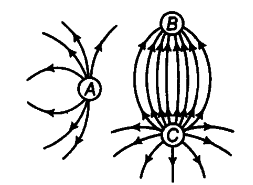# Figure shows the electric field lines

Figure shows the electric field lines around three point charges A, Sand C.(i) Which charges are positive?
(ii) Which charge has the largest magnitude? Why?
(iii) In which region or regions of the picture could the electric field be zero? Justify your answer.
(a) Near A (b) Near B © Near C (d) Near D

(i) Here, in the figure, the electric, lines of force
emanate from A and C. Therefore, charges A and C must be positive.
(ii) The number of electric lines of force emanating is
maximum from charge C here, so C must have the largest magnitude.
(iii) Point between two like charges where electrostatic force is zero is called neutral point. So, the neutral point lies between A and C only.Now, the position of neutral point depends on the strength of the forces of charges. Here more number of electric lines of force show higher strength of charge C than A. So, neutral point lies near A.